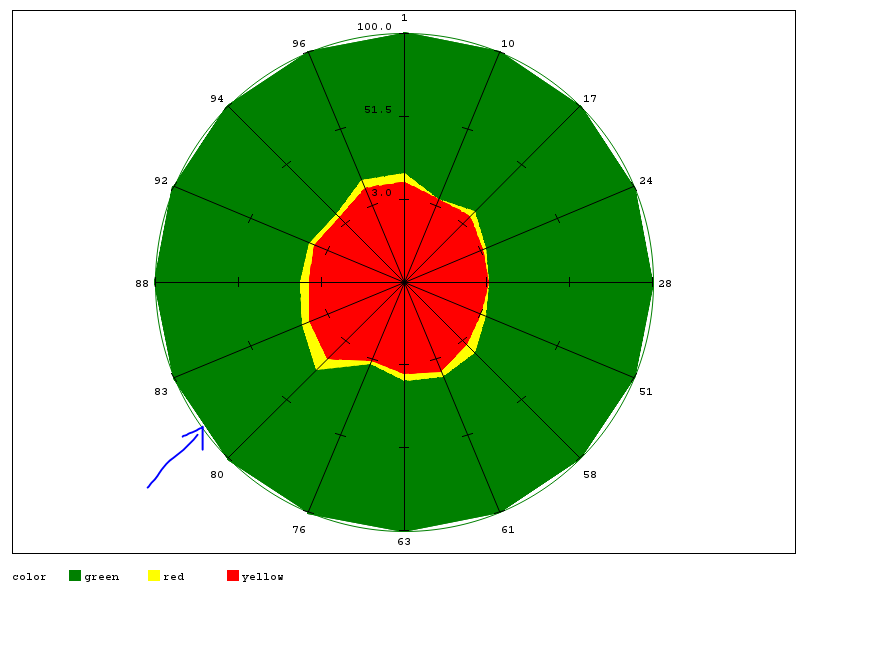Good day.

I'm using gradar proc and I trying to fill additional circle. I can't do anything with it.

This is my code.

```proc gradar data=test;
chart id /  freq=cnt
overlayvar=color
cstars=(green, yellow, red)
wstars=2
lstars=1
starcircles=(1)
cstarcircles=green
starfill=(solid solid solid)
cstarfill=( green, yellow, red)
run;
```

This is my problem:1 ACCEPTED SOLUTION

Accepted Solutions

You show a result but do not describe the "problem". I think you mean that you want the small section of arc filled in.

I think the easiest with this data would be to specify a WSTARCIRCLES=( ) option with a value larger than 1 for the first circle. Possibly WSTARCIRCLES=(3,1,1 )

2 REPLIES 2

You show a result but do not describe the "problem". I think you mean that you want the small section of arc filled in.

I think the easiest with this data would be to specify a WSTARCIRCLES=( ) option with a value larger than 1 for the first circle. Possibly WSTARCIRCLES=(3,1,1 )